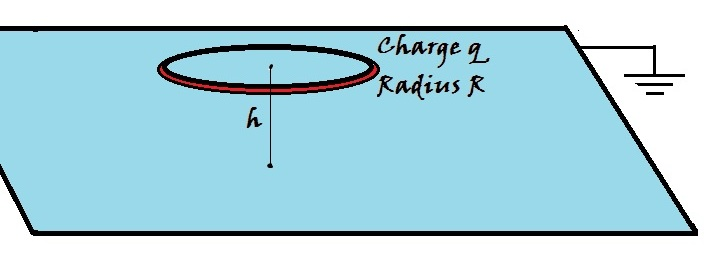# Charged Ring Over An Infinite Grounded PlaneA thin ring of radius $\displaystyle R$ is charged with a charge $\displaystyle q$. It is then placed over an infinitely large grounded conducting plane at a height $\displaystyle h$, as shown in the figure above.

Find the magnitude of the surface charge density $\displaystyle \sigma$ (in C/m$^2$) of the induced charge at the point on the plane which is exactly below the ring's center.

Details and Assumptions

$\bullet$ $\displaystyle q = 2\ \mu\text{C}$
$\bullet$ $\displaystyle R = 10\text{ cm}$
$\bullet$ $\displaystyle h = 15\text{ cm}$

×